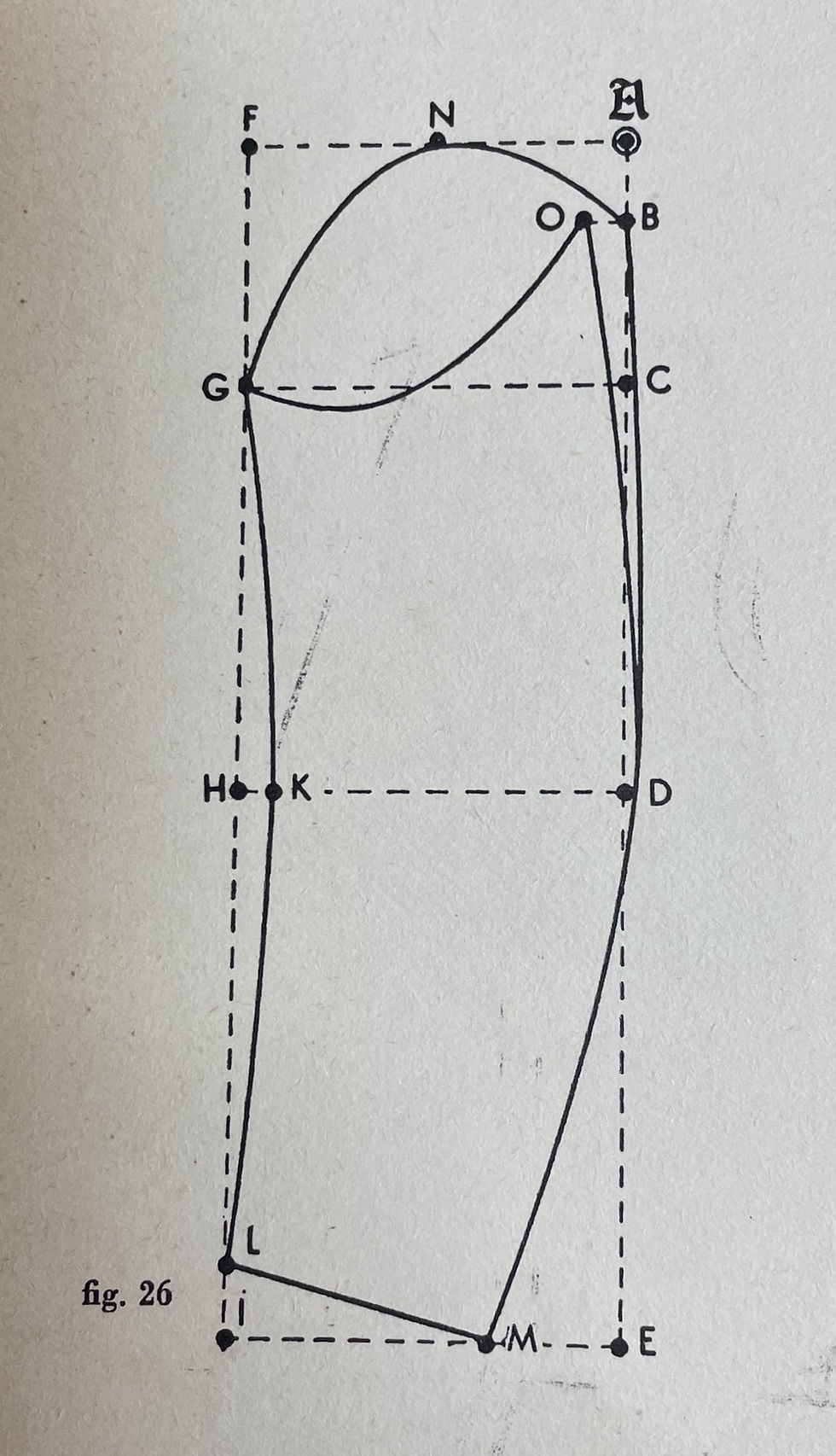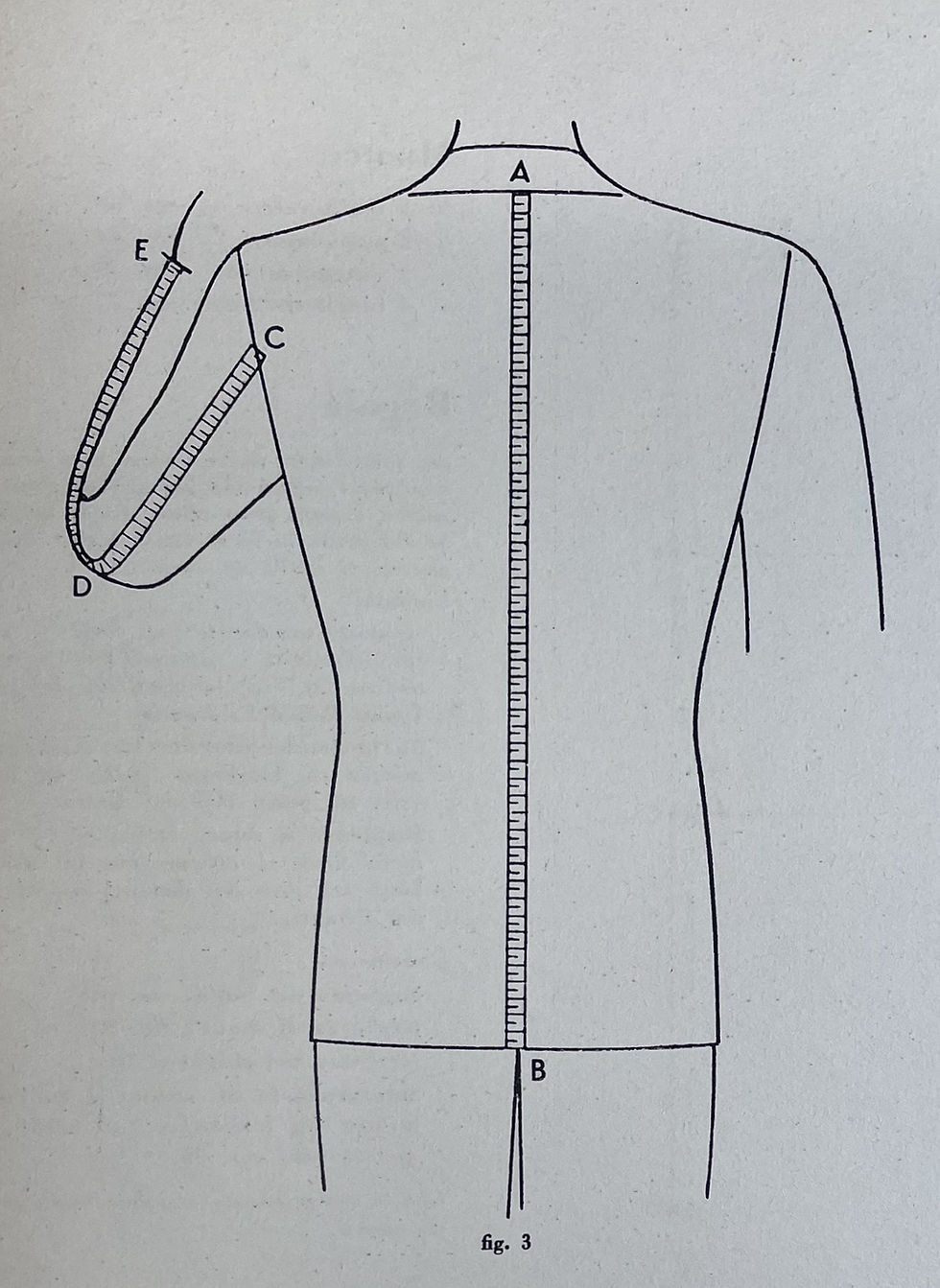top of page
Search
• ArtifexOfficial

# Ruggeri Cutting System

Updated: Jun 21, 2021Two-button SB Jacket

Measures Required:

1. Half-chest

2. Half-waist

3. Half-hip

4. Back waist length

5. Jacket length

Back-part

Point A: is a 90 degree angle;

Point B: is marked from point A using the measure of half of the half-chest (or 1/4 total chest). This a fixed rule from chest sizes of 32cm to 54cm.

Point C: is marked from point A with the measure of the back waist length;

Point D: is marked from point A with the measure of the jacket length. Points B, C and D are squared up from line A-D.

Point E: is marked from point B with the measure of 1/5 of the full chest measure;

Point F: is marked from point A with the measure of half B-E minus 1cm. A line is drawn vertically at 90 degrees from F.

Point G: is marked at 2.5cm on the vertical line from F;

Point H: is marked from point A with the measure of half A-F. A line starting from H is drawn parallel to that of B-E.

Point I: is marked diagonally from point G on the horizontal of H with the measure of half A-B plus 3cm (fixed quantity). This length represents the shoulder width. Connect points E and I with a line.

Point J: is marked from point I at 2.5cm (shoulder fullness);

Point K: is marked at 4-5cm from point E on the line E-I to form the underarm. From K a line is drawn parallel to B-E.

Point L: is marked from point C at 1.5cm (fixed measure);

Point M: is marked from point K to your liking or according to current styles (in this case 2.5cm);

Point N: is marked from point C with the measure of B-M minus 4cm or according to current styles;

Point O: is marked from point D with the measure of C-N plus 1.5cm or according to current styles;

Point P: is marked from point D with the same measure as C-L.

Complete the model as is shown in the graphic.Front-part

Extend the shoulder line of B-I from the back part across and square down. This right angle is point A.

Point B: is marked from A using the measure of B-H from the back-part;

Point C: is marked from point B using the measure of B-C from the back-part;

Point D: is marked from point C using the same measure of C-D from the back-part. Square across from points B, C and D.

Point E: is marked from point B using the same measure of B-E from the back-part;

Point F: is marked from point C with the measure of half of the half-waist (or 1/4 of full waist). Connect points E-F which will define the front edge of the jacket without the overlap.

Point G: is marked in the middle of B-E. Square up from G;

Point H: is marked from point G with the measure of half of the half-chest (1/4 of the full-chest) minus 1cm or (fixed rule from half-chests of 32cm to 54cm). Connect points E and H as well as B and H. E-H will serve as a reference for the neckline and B-H will serve as a reference for the armhole.

Point I: is marked diagonally from point H on the shoulder line using the shoulder width of G-I from the back-part (the shoulder line runs perpendicular to point A);

Point J: is marked from point B at 2.5cm (front sleeve notch);

Point K: is marked from point B with the measure of half E-B (chest width) that corresponds with 1/10 of the full-chest. Adding the measures of points B-E with the width of the armhole, points B-K, and with the shoulder width from the back-part, points B-E, results in the exact half-chest measurement.

Point L: is marked from point D using the measure of B-E;

Point M: is marked from point L using the half-hip measurement subtracted by the length of O-P from the back-part;

Point N: is marked from point M at 3cm for the seam allowance and ease;

Point O: is marked from point K at 3cm for the seam allowance and ease;

Point P: is marked at the intersection of of point C with line N-O;

Point Q: is marked from point O with the measure of E-K from the back-part plus 1cm;

Point R: is marked from point P at 1.5cm or according to current styles;

Point S: is marked from point E at 4-5cm;

Point T: is marked from point F at 4-5cm for the overlap;

Point U: is marked in the middle of points B-K;

Point V: is marked from point C with the measure of half B-U;

Point Z: is marked from point C at 9cm (pocket position).

Complete the model as is shown in the graphic.Sleeve

Point A: is a 90 degree angle;

Point B: is marked from point A at 4cm or to your liking. Square out from B;

Point C: is marked from point A using the same distance from points B-H from the back-part minus 4cm. This point can be fixed or modified depending on what type of sleeve head that is trying to be achieved.

Point D: is marked from point B with the elbow position;

Point E: is marked from point B with the total sleeve length. Square out from points C, D and E;

Point F: is marked from point A using the same distance of points B-H from the back-part plus 3-4cm. Square down from F. F-I and A-E are parallel;

Point G: is marked at the intersection of point F with point C;

Point H: is marked at the intersection of point F with point D;

Point I: is marked at the intersection of point F with point E;

Point K: is marked from point H at 2cm;

Point L: is marked from point I at 4cm (fixed measure);

Point M: is marked diagonally from point L at 15cm or according to current styles;

Point N: is marked in the middle of A-F;

Point O: is marked from point B at 2cm. This point, however, is regulated in the make of the garment from time to time according to the measure of the armhole (points I-J on the front-part and points J-M on the back part).

Complete the model as is shown in the graphic.

If you're uncertain as to how the measurements are taken, take a minute to review the following diagrams:Points A-B = back waist length

Points C-D = half-chest

Points E-F = half-waist

Points H-G = half-hipPoints A-B = jacket length

Points C-E = sleeve length

Points C-D = elbow position

A special thank you to Maestro Vittorio Coccurello of Sartoria Vittorio Coccurello for supplying this cutting system!

1,584 views

See All
bottom of page